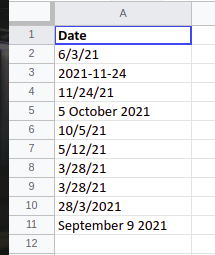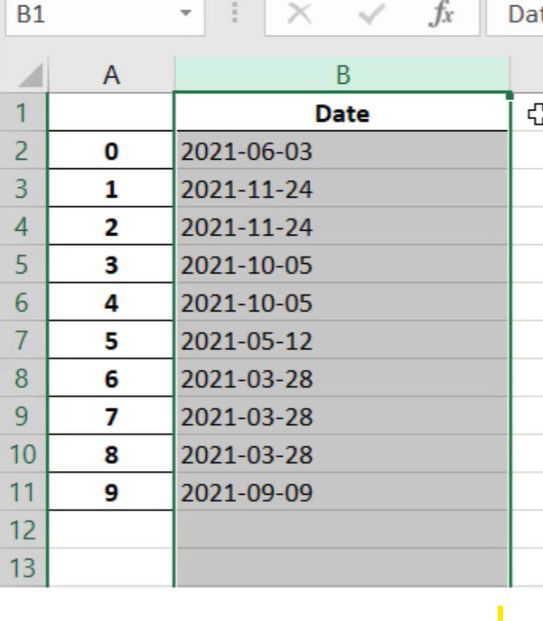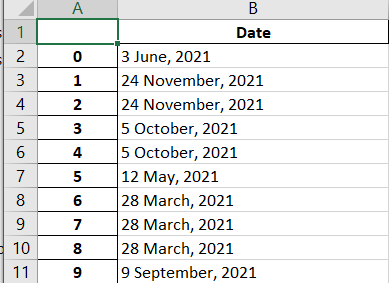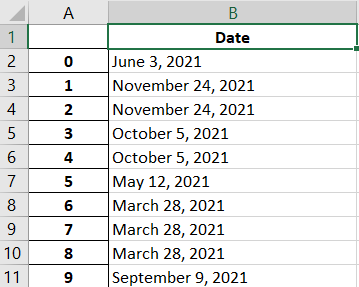# Convert any Dates in Spreadsheets using Python

• Last Updated : 07 Jan, 2022

In this article, we are going to see how to convert any Dates in Spreadsheets using Python.

Used file:This file comprises a single column entitled ‘Date’ and stores random dates of 2021 in some different forms of format.

### Approach:

• We’ll begin by importing the pandas library.
• Let’s have a look at the code that converts the dates.

sample_dates[“Date”] = pd.to_datetime(sample_dates[“Date”]).dt.strftime(“%Y-%m-%d”)

• To perform tasks involving date and time, we must first transform the column into a datetime data type, which this section of the code will do:

sample_dates[“Date”] = pd.to_datetime(sample_dates[“Date”])

• Then we use the dt and strftime methods with a value of “%Y-%m-%d” to inform Python how to format the date. Example here we used is “%Y-%m-%” where %Y is the full year, %m is the month with 2 digits and %d is the date with 2 digits.

## Python3

 `# This code converts any dates in spreadsheet`` ` `import` `pandas as pd`` ` `# Read the file and specify which column is the date``sample_dates ``=` `pd.read_excel(``"sample_dates.xlsx"``)`` ` `# Export output with dates converted to YYYY-MM-DD``sample_dates[``"Date"``] ``=` `pd.to_datetime(``    ``sample_dates[``"Date"``]).dt.strftime(``"%Y-%m-%d"``)``sample_dates.to_excel(``"sample_dates_formated.xlsx"``)`

Output:## Other well-known formats:

Example date – Saturday, 18 December, 2021, 7:00 PM

• “%A, %B %d” -> “Saturday, December 18”
• “%d-%b-%y” -> “18-Dec-21”
• “%d/%m/%Y” -> “18/12/2021”
• “%b %d, %Y” -> “Dec 18, 2021”

### Example 2:

We’re going to use the same

aset that we used in the last example.
`Format - "%d %b, %Y" -> "18 December, 2021"`

Here we will use ‘%#d’ to remove the padding of zero from the day, i.e. 08 to 8. This will not do padding of zero if the date is in single digit. We can use ‘%-d’ on Linux.

## Python3

 `# This code converts any dates in spreadsheet`` ` `import` `pandas as pd`` ` `# Read the file and specify which column is ``# the date``sample_dates ``=` `pd.read_excel(``"sample_dates.xlsx"``)`` ` `# Export output with dates converted to ``# "D MMMM, YYYY"``sample_dates[``"Date"``] ``=` `pd.to_datetime(``    ``sample_dates[``"Date"``]).dt.strftime(``"%#d %B, %Y"``)``sample_dates.to_excel(``"sample_dates_formated.xlsx"``)`

Output:### Example 3:

Here we will use different format

`Format - "%B %d, %Y" -> "December 18, 2021"`

## Python3

 `# This code converts any dates in spreadsheet`` ` `import` `pandas as pd`` ` `# Read the file and specify which column is``# the date``sample_dates ``=` `pd.read_excel(``"sample_dates.xlsx"``)`` ` `# Export output with dates converted to "MMMM D, ``# YYYY"``sample_dates[``"Date"``] ``=` `pd.to_datetime(``  ``sample_dates[``"Date"``]).dt.strftime(``"%B %d, %Y"``)``sample_dates.to_excel(``"sample_dates_formated.xlsx"``)`

Output:My Personal Notes arrow_drop_up